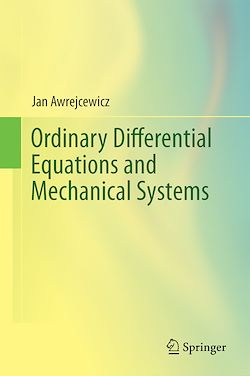# Ordinary Differential Equations and Mechanical Systems

-English
614 Pages

Description

This book applies a step-by-step treatment of the current state-of-the-art of ordinary differential equations used in modeling of engineering systems/processes and beyond. It covers systematically ordered problems, beginning with first and second order ODEs, linear and higher-order ODEs of polynomial form, theory and criteria of similarity, modeling approaches, phase plane and phase space concepts, stability optimization and ending on chaos and synchronization.

Presenting both an overview of the theory of the introductory differential equations in the context of applicability and a systematic treatment of modeling of numerous engineering and physical problems through linear and non-linear ODEs, the volume is self-contained, yet serves both scientific and engineering interests. The presentation relies on a general treatment, analytical and numerical methods, concrete examples and engineering intuition.

The scientific background used is well balanced between elementary and advanced level, making it as a unique self-contained source for both theoretically and application oriented graduate and doctoral students, university teachers, researchers and engineers of mechanical, civil and mechatronic engineering.

Subjects

##### Differential equation

Informations